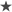Samsung TV model numbers use codes to describe the TVs. Once familiar with the codes you can learn a lot about a particular TV just from glancing at its model code.

Note that the below model codes refer to models sold in the UK. Please refer to your Samsung local website for information on model codes sold outside the UK.

Using model UE55F8000AFXZ as an example we can see that:

U = LED

E = Produced for Europe

55 = Screen size (in inches)

F = Manufactured in 2013

8000 = Series. This will differ depending on the features for that individual model. You can find out the specifications for a particular model by entering the model code into the search area on the Samsung website.

A = Features or design

FXZ = Manufacturing information

If you have a UE55F8000BFXZ instead of the UE55F8000AFXZ used in the example the difference between the two models would be a design change, such as a different coloured bezel, but all the other specifications would be the same.

See the charts below to see what the other codes mean.

TV Model Numbers

Example: UE55F8000AFXZ

Meaning:

U of UE55F8000AFXZ

Q = QLED

U = LED

P = Plasma

L = LCD

H = DLP

K = OLED

E of UE55F8000AFXZ

N = North America

E = Europe

A = Asia

55 of UE55F8000AFXZ

Size in inches

F of UE55F8000AFXZ

N = 2018

Q = 2017 QLED

MU = 2017 UHD

M = 2017 HD

KS = 2016 SUHD

KU =2016 UHD

K =2016

L = 2015

H = 2014

HU = 2014 UHD

F = 2013

E = 2012

D = 2011

C = 2010

B = 2009

A = 2008

8000 of UE55F8000AFXZ

This number designates the series. The exact specifications of each series will differ by model but generally the higher the series, the higher the specifications on that model.

A of UE55F8000AFXZ

Feature or Design e.g. this might designate a different stand

FXZ of UE55F8000AFXZ

Manufacturing information

Older models (2007 and earlier)

TV Model Numbers

Example: LN-T4681FX/XA

Meaning:

LN of LN-T4681FX/XA

FP or HP = Plasma

LN = LCD

HL = DLP

TX = CRT

T of LN-T4681FX/XA

Model year

T = 2007

S = 2006

R = 2005

P = 2004

N = 2003

M = 2002

46 of LN-T4681FX/XA

Size in inches

81 of LN-T4681FX/XA

Series

F of LN-T4681FX/XA

Resolution indicator (Full HD)

X of LN-T4681FX/XA